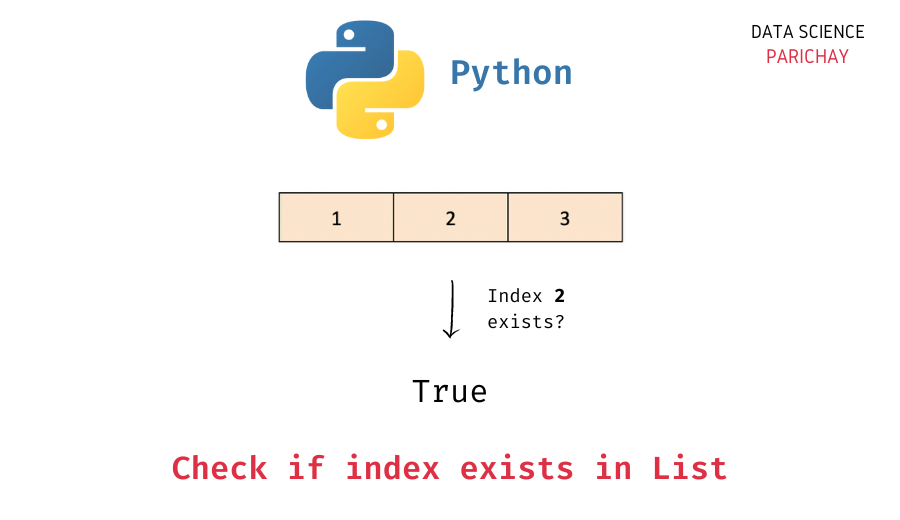# Python – Check If Index Exists in List

Lists are a very versatile data structure in Python that is used to store an ordered collection. In this tutorial, we will look at how to check if an index exists in a list or not.

Lists are mutable, so you can perform operations like adding elements, removing elements, extending a list, etc. The values in a list are indexed starting from zero and we can use a value’s index in the list to access it.

## How to check if an index exists in a list?

A Python list uses integer values starting from zero as its index. So, the first element in the list will have index 0 and the last element in the list has an index equal to the length of the list – 1 (one less than the length of the list).

If the index under consideration is less than the length of the list, we can say that the index exists in the list. The following is the syntax –

```# check if index exists
i < len(ls)```

We use the Python built-in `len()` function to get the length of a list. Here, we get `True` if the index exists in the list and `False` otherwise.

There are other methods as well that you can use to check if an index exists in a list or not. For example –

• Using `try` and `except` blocks. First, try to access the list value at the index under consideration inside a `try` block and use an `except` block to catch an `IndexError`. If the index exists in the list, no errors will be raised. If the index doesn’t exist, an `IndexError` is raised which will be handled by the `except` block (see the examples below).

## Examples

Let’s look at some examples of using the above methods.

### Example 1 – Check if an index exists using the list length

Let’s create a list and check if a valid index exists or not.

📚 Data Science Programs By Skill Level

Introductory

Intermediate ⭐⭐⭐

🔎 Find Data Science Programs 👨‍💻 111,889 already enrolled

Disclaimer: Data Science Parichay is reader supported. When you purchase a course through a link on this site, we may earn a small commission at no additional cost to you. Earned commissions help support this website and its team of writers.

```# create a list
i = 2
# check if index i is present in the list
print(i < len(ls))```

Output:

`True`

Here, we created a list of length 3 and checked for the index 2. You can see that we get `True` as the output as the index `2` exists.

Let’s now access an index that does not exist.

```# check if index 3 is present in the list
print(3 < len(ls))```

Output:

`False`

We get `False` as the output.

What if the list is empty? Let’s find out.

```# create an empty list
ls = []
# check if index 0 is present in the list
print(0 < len(ls))```

Output:

`False`

Here, we created an empty list and checked if the index `0` is present. We get `False` as the output.

### Example – Check if an index exists using `try` – `except`

Alternatively, we can use error handling to check if an index exists in the list or not.

The idea is to try to access the list value at the given index inside a `try` block, now if the index exists, no errors will be raised but if the index does not exist, an `IndexError` gets raised which can be handled by an `except` block.

Let’s look at an example.

```# create a list
i = 3

# check if index i is present in the list
try:
ls[i]
print("Index present")
except IndexError:
print("Index not present")```

Output:

`Index not present`

Here, we tried accessing the element at index 3 in a list with three values. Now, since index 3 does not exist, an `IndexError` got raised which was then handled by the `except` block. Thus, you can see the result, “Index does not exist”.

## Summary

In this tutorial, we looked at some methods to check if an index exists in a Python list or not. The following are the key takeaways –

• If the index is less than the length of the list you can say that the index exists in the list.
• Alternatively, you can use error handling to check if an index exists or not. If an `IndexError` gets raised on trying to access the value at the given index, you can say that the index does not exist.

You might also be interested in –

## Author

•Piyush is a data professional passionate about using data to understand things better and make informed decisions. He has experience working as a Data Scientist in the consulting domain and holds an engineering degree from IIT Roorkee. His hobbies include watching cricket, reading, and working on side projects.

Scroll to Top